# Cosmic ray and solar energetic particle flux in paleomagnetospheres

## Abstract

The geomagnetic field is part of the shield prohibiting energetic particles of solar and cosmic origin directly hitting the Earth surface. During geomagnetic polarity transitions the geomagnetic field strength significantly decreases with energetic particles having a much better access to the atmosphere and surface. To study in more detail the flux of energetic particles into the paleomagnetosphere we use a potential field approach to model the paleomagnetosphere which generalizes the parametric model of Voigt (1981) by taking into account a non-zero quadrupole moment of the core field. We study in particular the quadrupolar situation as a geomagnetic polarity transition is also characterized by a significant increase of non-dipolar contributions. Our model is used as a tool for tracing particle trajectories in such paleomagnetospheres and to assess variations of high-energy particle fluxes into the atmosphere. As a first application of the particle tracing scheme we determine cutoff latitudes and impact areas for different paleomagnetospheric configurations. For configurations with equivalent magnetic field strength or magnetic energy the impact area is very similar.

## 1. Introduction

Planet Earth possesses a global magnetic field since at least 3.2 billion years (Tarduno et al., 2007). The Earth’s magnetosphere results from the interaction of the solar wind with this internally generated planetary magnetic field. Variations of the internal magnetic field provoke changes of the magnetospheric field and also of the size of the magnetosphere. During geomagnetic polarity transitions the dipole field orientation not only reverses, but the field strength at the Earth’s surface significantly drops. Furthermore, non-dipole contributions, especially quadrupolar field contributions determine the field topology (Leonhardt and Fabian, 2007).

During a polarity transition the magnetosphere is significantly altered. Siscoe and Chen (1975) and Saito et al. (1978) coined the term ‘paleomagnetosphere’ for these magnetospheric situations. The question emerges whether energetic particles of solar and cosmic origin have much better access to the terrestrial atmosphere under paleomagnetospheric conditions and how they impact the terrestrial biosphere under polarity transition conditions.

A first step in answering this question is a detailed analysis of the topology of the magnetospheric magnetic field during a polarity transition. There are different ways to examine the magnetospheric magnetic field in dependency of the internal one. One is to use MHD simulations; another method is to rely on quantitative modeling. The first method gives a detailed view of the magnetic field topology within the magnetosphere. Vogt et al. (2004, 2007) simulated magnetospheres with different internal magnetic field, dipoles as well as quadrupoles. MHD simulations allow to study reconnection effects and the resulting magnetic field topology particularly in the outer magnetosphere where the magnetic field is relatively weak. This region is expected to affect energetic particles of solar origin with energies in the MeV range and relatively small gyro radii that tend to follow the field lines (Vogt et al., 2007).

The orbits of particles at typical cosmic ray energies in the GeV range, however, are influenced mainly by the inner magnetosphere where the magnetic field is strong, and where MHD simulations are less helpful because of their limited spatial resolution. Here quantitative parametric models are not only less expensive in terms of computational resources, they also resolve the magnetic field geometry in sufficient detail to study cosmic ray orbit connectivity. Therefore, in the present paper we describe an efficient way to determine magnetospheric models suitable for the calculation of energetic particle trajectories under the conditions of a geomagnetic polarity transition. Our calculations apply to galactic cosmic ray events and the relatively isotropic phase of solar cosmic ray events.

Quantitative models of the external geomagnetic field (e.g. Mead and Fairfield, 1975; Voigt, 1981; Tsyganenko, 1990; Jordan, 1994; Stern, 1994; Hilmer and Voigt, 1995; Siscoe, 2001) are widely used in the magnetospheric community for various purposes. At least two different modeling strategies can be distinguished. Empirically oriented models (e.g., Alexeev, 1978; Stern, 1985; Tsyganenko and Usmanov, 1982; Tsyganenko, 1987, 1989, 2002) are constructed by minimizing the discrepancy between the model output and satellite observations. These models describe the present magnetosphere extremely well. A second class of models (e.g. Voigt, 1981; Hilmer and Voigt, 1995; Romashchenko and Reshetnikov, 2000; Willis et al., 2000) makes use of a potential field approach to model the magnetic effect of the Chapman-Ferraro currents on the magnetopause. For brevity, we refer to the latter type as ‘potential field models’. Since potential field models are constructed on the basis of the shape of the magnetopause and the parameters of the internal field rather than an empirical data set, they can be used to assess magnetospheric configurations which differ significantly from today’s Earth’s magnetosphere. For example, the potential field approach was successfully used to model the magnetospheres of other planets (Voigt et al., 1987; Voigt and Ness, 1990). Scaling relations which can be used to adapt quantitative magnetospheric models to the case of smaller or larger values of the Earth’s dipole moment were discussed by Vogt and Glassmeier (2001), Glassmeier et al. (2004), and Zieger et al. (2006).

In order to investigate how higher-order multipoles affect the magnetospheric configuration and particle trajectories, we generalize the 3-dimensional parametric model developed by Voigt (1981) to include quadrupole contributions to the internal field. The shape and the size of the magnetopause are given: we assume the model magnetopause to consist of a cylindrical magnetotail topped by a half-sphere representing the dayside magnetopause. The parameters entering the model, the magnetic moments and the size of the magnetosphere, can be varied independently of each other because of the assumed fixed geometry. Note that earlier quantitative models of quadrupolar magnetospheres were either two-dimensional models (Biernat et al., 1985; Leubner and Zollner, 1985; Starchenko and Shcherbakov, 1991) or highly (spherically) symmetric models (Willis et al., 2000).

Of particular interest in paleomagnetospheric studies is the motion of charged particles in different magnetospheric configurations. Since the magnetosphere acts as a kind of filter for charged energetic particles, polarity transitions are supposed to strongly affect their flux into the upper atmosphere. Solar proton events can cause ozone depletion (e.g. Stephenson and Scourfield, 1992; Jackman et al., 2005). Galactic cosmic rays may have an influence on cloud coverage, which in turn influences the global radiation budget and thus the climate (Svensmark and Friis-Christensen, 1997; Marsh and Svensmark, 2000). Trapped particle populations and ring current geometries in quadrupolar magnetospheres were studied by Vogt and Glassmeier (2000). The scaling behavior of different classes of energetic particles in dipolar and quadrupolar paleomagnetospheres was investigated by Vogt et al. (2007).

The parametric model developed here is designed to be used for the integration of high-energy particle orbits of solar and cosmic origin in a dipole-quadrupole paleomagnetosphere. The computationally efficient evaluation of the model magnetic field allows to trace a large number of particles. The resulting upper atmospheric flux function constitutes the direct interface to research groups working on paleonucleotide production and ozone depletion (e.g. Quack et al., 2001; Sinnhuber et al., 2003; Winkler et al., 2008). The parametric approach presented here is complemented by magnetohydrodynamic simulations published elsewhere (Vogt et al., 2004; Zieger et al., 2004, 2006). MHD simulations treat the magnetopause and the tail region self-consistently and thus can in principle provide a more realistic picture of the global magnetospheric configuration. They are, however, computationally more expensive, and their spatial resolution is limited in particular in the inner magnetosphere which has strong influence on cosmic ray trajectories. The parametric approach pursued here does not suffer from these constrains. Thus, we prefer the parametric approach.

This paper is organized as follows. In Section 2 the approach for the magnetic field model is presented and details of the mathematical description are given. The calculation of particle trajectories and the results of cutoff latitudes and impact areas are described in Section 3. Section 4 deals with some applications of the model. We consider different dipole and quadrupole configurations. For some of these configurations particle impacts on the atmosphere will be shown. The results will be summarized and discussed in the light of constraints from paleomagnetism in Section 5.

## 2. Magnetic Field Modeling Approach and Mathematical Description

The basic idea and the assumptions of the magnetospheric model used have been developed by Voigt (1976, 1981). The model is extended to a more general case by including a quadrupolar contribution to the internal magnetic field of the Earth.

### 2.1 Internal field and external current systems

The magnetospheric magnetic field of the Earth is the result of a superposition of an internal and an external part. The internal magnetic field e is generated by hydromagnetic processes in the liquid core of the Earth (e.g. Wicht et al., 2009). The field on the Earth’s surface can be described by a multipole field (e.g. Gauss, 1839; Chapman and Bartels, 1962). Voigt (1976, 1981) considered only a dipole moment, because the most dominant part of the internal Earth’s magnetic field at the present time is dipolar. In the generalization presented in the following sections the magnetic field will be represented by a dipole moment m̱ and a quadrupole moment . Both moments are centered within the Earth.

In general the external magnetic field is caused by current systems, mainly consisting of the equatorial ring current, the tail currents and the magnetopause currents, i.e. the Chapman-Ferraro currents (Jordan, 1994). In the far field the magnetic field of the ring current can be modeled like a dipolar field (Stern, 1985). To get a simple approximation for the magnetic field line topology in the magnetosphere, the inner dipole field and the ring current field are combined to a magnetic field of a modified dipole moment. Vogt and Glassmeier (2000) showed that in quadrupole magnetospheres the configuration and location of the ring current may be totally different from the present situation. In some cases one cannot even speak of a ring current any more. Instead of modeling the tail currents explicitly Voigt (1981) stretches the field lines on the nightside into the far tail. In the extended model presented in this paper, this feature had also been implemented but it did not yield significant effects on particle trajectories in the energy range considered here, so it will not be described in the following text.

In our magnetospheric model only the magnetopause currents will be treated. The magnetic field cf caused by Chapman-Ferraro currents shields the Earth’s magnetic field against the interplanetary magnetic field. In a closed magnetosphere no field lines penetrate the boundary of the magnetosphere, the magnetopause. Thus, the normal component of the total magnetic field has to vanish on the magnetopause. The total magnetic field is constructed by superposing the Earth’s internal field e and the field of the Chapman-Ferraro currents cf: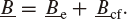(1)

### 2.2 Model geometry

The magnetosphere is not self-consistent but it has a prescribed geometry (see Fig. 1). Its shape is represented by a half-sphere with a radius Rm on the dayside and semi-infinite cylinder with the radius Rm on the nightside. Both are matched continuously to each other. The Earth is placed within the half-sphere.

The two coordinate systems used in the following calculations are shown in Fig. 1. One is the well known geocentric-solar-ecliptic (GSE) system, where xg is towards the sun, xg and yg span the ecliptic plane and zg is perpendicular to the other ones. The Earth is in the center of the system. Because of the special geometry of the magnetosphere, we introduce a second coordinate system, the so called M(odel)-coordinate system (x, y, z), which is more convenient for the following calculations. Its origin is located in the center of the connecting plane between half-sphere and cylinder, which is also the center of the half-sphere. The z-axis coincides with the axis of the cylinder. The transformation between these two coordinate systems is given by(2)

Due to the different geometries on the dayside and in the tail, in the M-coordinate system not only Cartesian, but also spherical (r, ϑ, λ) and cylindrical (ρ, ϑ, z) coordinate representations are used.

Several parameters, which can be varied independently, define the geometry and the magnetic field configuration of the magnetospheric model: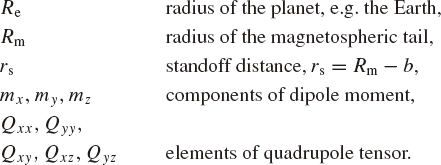SI-units are used throughout this paper. The components of dipole and quadrupole moments are given in the Cartesian GSE-coordinates. There are only five-independent quadrupole components, because is a symmetric tensor with Trace ()=0.

The standoff distance is the distance from the center of the planet to the subsolar point on the magnetopause. As already mentioned the magnetosphere is a result of the interaction of the solar wind with the internal planetary magnetic field. The magnetic moments characterize the internal magnetic field, whereas the standoff distance rs and the radius of the magnetospheric tail Rm are determined by a pressure equilibrium between the solar wind pressure and the magnetic pressure of the planetary field on the magnetopause (Mead and Beard, 1964; Starchenko and Shcherbakov, 1991). For a nearly zonal dipole, corresponding to the present magnetic field configuration of the Earth, Siscoe and Chen (1975), Glassmeier et al. (1984, 2004), and Vogt and Glassmeier (2001), give some scaling relations, which can be used to get the standoff distance and the radius of the magnetospheric tail for different strength of the magnetic dipole moment. In order to determine the two magnetospheric parameters for other configuration, like pole-on or quadrupoles, we use the pressure equilibrium mentioned above. Our calculations fit quite well with the results of the MHD simulations of Vogt et al. (2004, 2007).

### 2.3 Potential field approach and boundary conditions

Since the current system is reduced to the Chapman-Ferraro currents on the magnetopause, there are no currents within the magnetosphere. In addition, the magnetic field model is supposed to be a static one, so all time derivatives vanish. With this the magnetic field can be derived from a scalar potential Φ: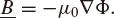(3)

With Maxwell’s equation ·Ḇ=0 we derive the Laplace equation for the scalar potential,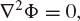(4)

which has to be solved using the following boundary conditions.

The Chapman-Ferraro currents and the formation of the magnetopause are associated with the frozen-flux theorem for ideal MHD flows which suggests that the interplanetary magnetic field lines do not connect to the terrestrial field lines. Hence, as a first approximation, the magnetosphere can be considered as closed which implies that the normal component of the total magnetic field must vanish on the magnetopause: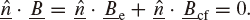(5)

A second boundary condition is that the magnetic field should vanish at infinity, in this case at the open end of the cylinder: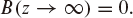(6)

The third boundary condition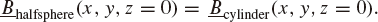(7)

describes the continuity of the potential at the connecting plane between the half-sphere and the cylinder.

### 2.4 Solution strategy

The internal magnetic field can be deduced from a scalar potential Φe. It will be described by a superposition of a dipole and a quadrupole potential (Jackson, 1963):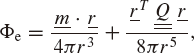(8)

where T is the transposed vector of .

The external magnetic field is caused by the Chapman-Ferraro currents. Here we do not intend to model the currents themselves but only the resulting magnetic fields. The magnetospheric magnetic field is determined in the following way: For a given internal magnetic field e the normal component on the magnetopause is calculated.

In order to satisfy the boundary condition (5) an additional magnetic field cf is constructed in such a way that the normal component of the superposed magnetic field =e+cf vanishes on the magnetopause. Since we do not consider any currents outside the magnetopause, the field cf caused by the Chapman-Ferraro currents can be derived from a scalar potential.

To find an analytical solution for the magnetic field e on the magnetopause and for the construction of the additional field cf, the calculation has to be split in one part for the half-sphere and another part for the cylinder.

#### 2.4.1 Solution on the dayside magnetosphere

The calculation in the half-sphere of the dayside magnetosphere will be done in spherical M-coordinates. Therefore the potential of the internal magnetic field of the planet, given in Eq. (8) has to be transformed from the Cartesian GSE-coordinate system (xg, yg, zg) into the Cartesian M-coordinate system (x, y, z) using Eqs. (2):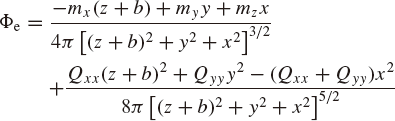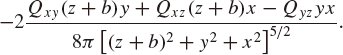(9)

Since a spherical geometry is used on the dayside, a further transformation into spherical coordinates is required: x = r sin θ cos λ, y = r sin θ sin λ, z = r cos θ. And the terms will be sorted by the order of harmonics: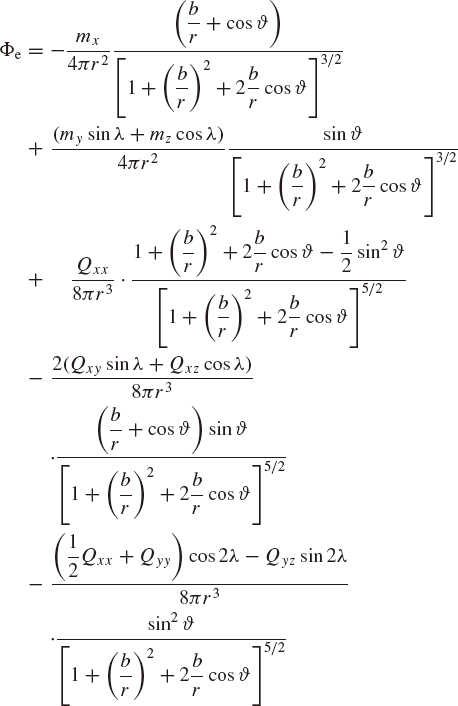(10)

so that they can be expanded into spherical harmonics using the generating function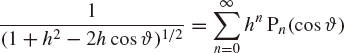(11)

and its derivatives; here $$h = - \frac{b}{r}$$. The associated Legr endre’s polynomials P m n =P m n (cos ϑ are not normalized. Thus we obtain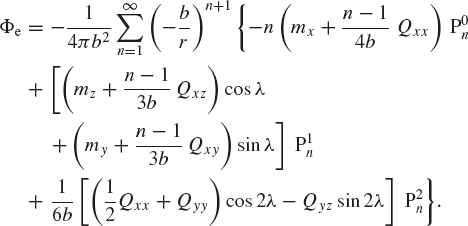(12)

As discussed above the magnetic field cf of the Chapman-Ferraro currents can be represented by a scalar potential cf, and the following ansatz is used (Voigt, 1981):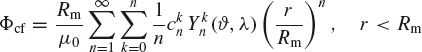(13)

where Y k n (ϑ, λ) denotes spherical harmonics. To satisfy the the boundary condition (5) we require: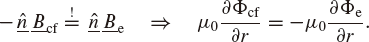(14)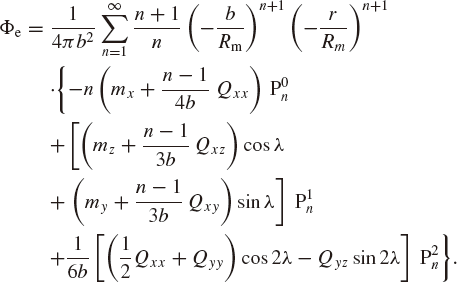(15)

The magnetic field on the dayside magnetopause then is given by the superposition of the fields, that is the sum of Eqs. (9) and (15):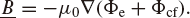(16)

#### 2.4.2 Solution in the tail

In the magnetospheric tail the calculations are done in cylindrical coordinates. One solution of the Laplace equation (4) in cylindrical coordinates considering the boundary conditions (5)–(6) are Fourier-Bessel series (Voigt, 1981). Therefore the following ansatz is used for the total potential in the tail: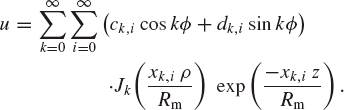(17)

In order to determine the coefficients of this series, the boundary condition (7) has to be used. If the potential is continuous, two components of the magnetic field, i.e. B p and , are continuous, too, B z not. This results from the fact, that due to the two different coordinate systems the Laplace equation (4) is not separable in the whole magnetosphere (Voigt, 1981). To solve this problem, i.e. to achieve continuity also for B z , Voigt (1972) developed an iterative process to determine proper coefficients; for further details see his explanations. The initial values of the coefficients are determined by the continuity of the potential on the connecting plane between the half-sphere and the cylinder.

Thus the total potential Φ = g F+ Φcf will be transformed into cylindrical M-coordinates. Now Φ* := Φ(z = 0) is calculated with z = cos ϑ = 0, ρ = r and ϕ = λ The radial dependence in the potential cf will be represented by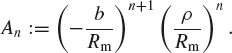(18)

After sorting the terms by the harmonics one obtains: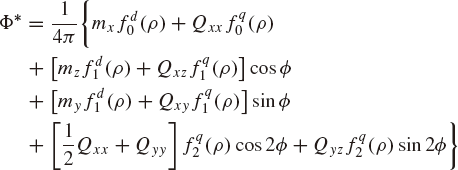(19)

with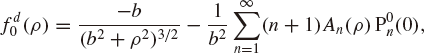(20)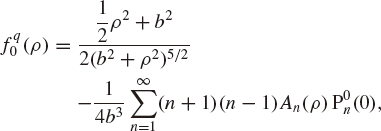(21)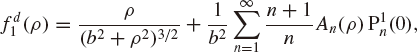(22)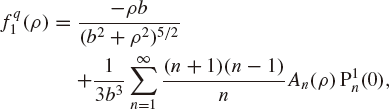(23)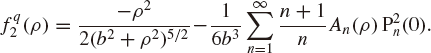(24)

The functions ƒ d/q k are radial functions, which can be expanded into Fourier-Bessel series: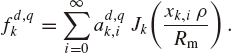(25)

The coefficient k represents the order of the harmonic of the trigonometric function. Here k only takes on values k = 0, 1, 2 as we are using dipole and quadrupole only. The quantity x k,i is the i-th root of the derivative of the Bessel function $$\frac{d}{{{d_x}}}{J_k}\left( x \right){|_{{x_{k,i}}}} = 0$$. The coefficients a d,q k,i can be calculated from the orthogonality relations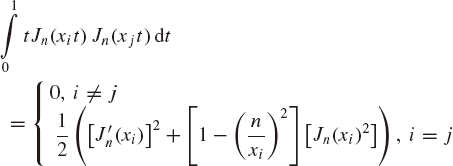(26)

and we obtain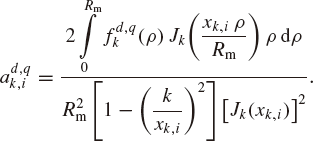(27)

Comparing Φ* from Eq. (19) with Eq. (17) at the location z =0 gives the solution for the potential Φ in the cylinder: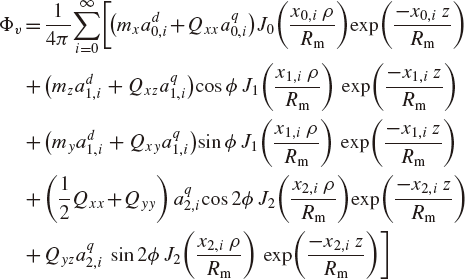(28)

The magnetic field in the tail can now be expressed as Ḇ=-μ0Φ;. The model was validated in several ways, e.g., by checking if the boundary values are consistent with the imposed conditions, and through comparison with published results for magnetospheric fields of other planets. The latter has been done by calculating the magnetospheric magnetic field of the Earth (Hilmer and Voigt, 1995) and of the planet Neptune (Voigt and Ness, 1990).

### 2.5 Generalization to higher-order multipoles

In a similar way, using the same procedure as in Section 2.4 higher-order multipoles of the internal magnetic field of the Earth can be added. A general expansion of higher-order multipoles in Cartesian coordinates is given by Lense (1954):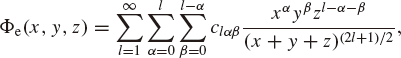(29)

where l is the order of the multipole. For each multipole there are (l + 1)(l + 2)/2 coefficients c lαβ . Only 2l +1 of them are linearly independent. The dependency can be determined by 2Φe =0.

This ansatz can be used instead of Eq. (8) or (9). After transformation to spherical model coordinates this equation, corresponding to Eq. (12), contains Legendre’s polynomials P m l (cos ϑ) with m = 0…l. However, in the present work we only make use of dipole and quadrupole moments.

## 3. Cosmic Particles in Possible Paleomagnetic Fields

### 3.1 Calculation of particle trajectories, cutoff latitudes and impact areas

Using the magnetospheric model described above the magnetic field can be determined at any location inside the magnetosphere and can be used for further applications like the calculation of particle trajectories and particle impacts on the atmosphere of the Earth.

In order to quantify the impact of particles on the Earth’s atmosphere cutoff latitudes and impact areas are determined. The cutoff latitude is defined by that latitude which divides regions where particles reach the Earth from those where no particles are found. This quantity is quite descriptive for symmetrical magnetic field configuration like the zonal dipole (Smart et al., 2000).

For other configurations we introduce the concept of “impact area” that should not be confused with the term “impact zone” as used by Firor (1954). Based on the idea that a particle of a given rigidity starting at a well-defined point source gives rise to an impact point of the respective trajectory on the Earth’s surface, Firor (1954) studied groups of impact points that originated from a source region of finite size, and termed such groups “impact zones”. The term “impact area” can be understood as a generalization of that earlier concept. In the present study, impact area quantifies the portion of the planetary surface that is accessible to particles of a given energy, relative to the total surface. Impact area is given as a percentage value. To determine cutoff latitudes and impact areas, a large number of trajectories are numerically integrated in the magnetic field configuration of interest.

The trajectories start on a shell with the radius rRm, i.e. outside the magnetosphere. We use a homogeneous distribution for the location on the this shell and for the angle between the normal vector on the shell and the velocity vector. More specifically, several thousand (typically 5400) different starting positions on the outer shell are defined. For each energy and at each initial position, about thousand (typically 1350) trajectories are launched with different initial directions (angles in velocity space). The trajectory itself is calculated using the numerical Leapfrog method (Birdsdall and Langdon, 1985). All particles entering the atmosphere which is described by a spherical shell hundred kilometer above the Earth’s surface, i.e. Ra = Re + 100 km, are counted and the particle impact density as well as the cutoff latitudes are determined. The particle fluxes considered here do not change much from this shell to the Earth’s surface. Thus for brevity and simplicity, we do not strictly distinguish these two shells (with the radii Re and Ra)in the following discussion. Results for the cutoff latitudes and impact areas are shown in Figs. 4–7.

The tracing routines are verified by comparing them with analytical results of charged particles in a homogenous magnetic field, and with particle trajectories calculated by Willis et al. (1997). Note that the orbit tracing process is reverse of that in the cosmic ray literature where the orbits start near Earth and are integrated outwards. Cosmic ray energies are typically in the GeV range or even above but in our case also solar energetic particles in the MeV range had to be considered (the simulation code was designed to cover energies down to 1 MeV). At lower energies, the efficiency of the outward orbit tracing approach suffers from the increasing proportion of re-entrant particles (trajectories with both start points and end points near Earth) that do not contribute to the energetic particle flux of solar or cosmic origin but simply produce computational overhead. The main problem associated with inward orbit tracing is the class of trajectories that miss the planet but this particle population is easier to control, and in our case it produced less computational problems than the returning particle population near the planet.

Furthermore, the cosmic ray community frequently uses particle rigidity (momentum per charge) to study cutoff parameters and asymptotic directions. This is important in the case of cosmogenic data because almost 50% of the cosmogenic nuclides in the atmosphere are produced by alpha particles and heavier cosmic rays (McCracken, 2004). At identical rigidity values, protons and alphas follow the same trajectories whereas identical kinetic energies in general yield different orbits. More specifically, for a given rigidity, it is the ratio of charge and atomic mass that determines the cutoff energy. This ratio is unity for a proton but approximately 0.5 for all other fully ionized nuclei, so we can consider alpha particles as representatives for the latter class of cosmic rays. The relationship between rigidity and kinetic energy is discussed in more detail by McCracken (2004) and by Vogt et al. (2007). For the proton kinetic energy levels used in this study, the associated rigidity values and kinetic energies of alpha particles are given in Table 1 and Fig. 2.

We also note that the cutoff parameters given in Section 4 are determined on the basis of all incident trajectories, i.e., irrespective of their angles of approach. These parameters should be distinguished from the vertical cutoff parameters that take into account only trajectories with zero zenith angle of approach. Hence our cutoff energies belong to incident angles where the trajectories are least constrained by the magnetic field configuration. For the present-day dipole field, this is a zenith angle of 90 degrees from the geomagnetic west. At this incident angle, the Størmer cutoff energy at the (geomagnetic) equator is about 10 GeV whereas the vertical Størmer cutoff is about 14 GeV.

Smart and Shea (2001) computed geomagnetic cutoff rigidities and latitudes in an empirical Earth magnetic field using the Tsyganenko magnetospheric field model. Cutoff latitudes determined by our simple model yield similar results. Thus the model presented in Section 2 is a reasonable tool to determine the main characteristics of particle fluxes in the present magnetosphere. And therefore we assume that it works also rather well in the case of paleomagnetospheres. In Section 4 we examine particles with energies of 64 to 8192 MeV in magnetic fields of g m n = 5000…3000 nt.

### 3.2 Scalability of the model and the trajectories

One advantage of the analytical model developed above is the possibility to scale the geometrical size of the magnetosphere by changing the size of the magnetic moment. Therefore three non-dimensional coordinates r/Rm in the half-sphere, ρ̃ρ/Rm and z/Rm in the cylinder, and two scaling parameters c1:= p/p0, where p denotes either the dipole moment or the quadrupole moment, and c2 = Rm,0/Rm were defined. The scaling parameters describe the ratio between the present day configuration, subscribed by the index 0 and any other magnetic configuration. Note that rs/Rm = const. Introducing these parameters, each component B i of the magnetic field can be written as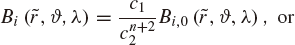(30)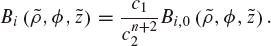(31)

Keep in mind that n = 1 in case of a dipole and n = 2 for a quadrupole configuration. We focus on magnetospheric variations induced by the internal field and disregard possible changes of the solar wind conditions. Therefore the magnetic field on the magnetopause should be the same field 0 as in the present time as the magnetic pressure has to balance the solar wind pressure. Thus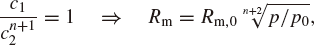(32)

e.g. for a magnetic dipole moment one tenth of the present day value, the magnetospheric radius and the standoff distance decreases approximately by a factor of two.

Note that this scaling is only valid for pure dipole or pure quadrupole configurations, but not for mixed cases. For a zonal dipole configuration the scaling relation can already be found in e.g. Siscoe and Chen (1975) or Vogt and Glassmeier (2001).

Using the non-dimensional coordinates all magneto-spheres of the same multipole configuration but with different strength can be normalized: The topology of the field lines are the same for all values of the magnetic moments, but the normalized size of the Earth differs. For small moments it is larger than for large ones. Figure 3 shows an example of two magnetospheric situations. The left upper figure demonstrates the magnetic field lines of a magnetic dipole moment mz,0 in a cylindrical magnetosphere, the right one shows the magnetic field of a moment mz,1 = 0.1mz,0. The size of the magnetosphere, determined by Eq. (32), is about half that of the first case. Zooming the first magnetosphere by a factor of one and the second by a factor of two, as seen in the lower figure of Fig. 3, the field lines are the same, but the radius r1 of the sphere, which describes the Earth with the lower dipole moment is twice as large as the radius r0 for the case with the larger dipole moment.

Like the magnetic field also the particle trajectories are scalable. From the point of view of computing time this is interesting especially for calculating particle trajectories in different paleomagnetic field configuration. In order to determine different particle impacts on the Earth trajectories are calculated for each configuration, e.g. m z or mz,0 , and particles reaching the Earth are counted. Using now the scalability of the model only one trajectory for all configurations together needs to be calculated instead of one trajectory for each configuration. The counting of particles reaching the Earth is now done on several spheres (r0 and r1) instead of one sphere representing the Earth in the unscaled case.

## 4. Model Application: Possible Magnetic Field Con.gurations and Cutoff Angles During Reversals

Paleomagnetic measurements of sediments and lava rocks show variations of the magnetic field of the Earth on very long time scales. The most dramatic variation is a complete reversal of the magnetic field, which occurred roughly every 105–106 years. The mechanism of these reversals is not well understood yet, but see Wicht et al. (2009) for further details. From paleomagnetic measurements it is known that the magnetic field decreases and changes the direction during a reversal. Merrill and McFadden (1999) point out several reversal models and bring up different magnetic field configuration during polarity transitions, which include dipoles as well as higher multipoles. Some dipole and quadrupole configurations we will present in the next section.

### 4.1 Dipole configuration

Saito et al. (1978), for example, proposed a turning dipole scenario. During this kind of reversal the tilt angle grows and the magnetospheric configuration passes the pole-on configuration. At the same time the strength of the magnetic dipole decreases. Afterwards the tilt angle increases until the dipole moment is antiparallel to the present one. The magnetic dipole strength increases in the meantime. In the following we will discuss two possible dipole configuration with different strengths.

In an idealization of the present day magnetospheric configuration the dipole axis (as well as the rotation axis) is perpendicular to the line connecting the sun and the planet (which is the x-axis in GSE-coordinates). This is shown in Fig. 4(a). It corresponds to a nonzero Gauss coefficient g 01 of a spherical expansion of multipoles (while all other coefficients vanish).

Cusp regions are found in the polar regions on both hemispheres. Magnetic field lines from the dayside are deected to the nightside and they are stretched into the deep tail. Charged particles experience the Lorentz force which acts perpendicular to the magnetic field lines but not parallel to it, thus they can more easily reach the Earth’s surface in the polar regions where the field lines are radial. Figure 4(b) displays the regions where high energetic particles (4 GeV) penetrate to Earth in a magnetic field strength of the present (i.e. g 01 = 30000 nT). The hatched areas are those where none of these particles are detected.

The solid line in Fig. 4(c) shows the cutoff latitudes of a zonal dipole of the present strength for different particle energies. The cutoff latitude is defined by that latitude which divides regions with and without particle impacts, as seen in Fig. 4(b). Thus, particles reach the Earth in regions ϑcutoffϑ ≤ 90°, where ϑcutoff is the cutoff latitude. The result agrees reasonably well with calculations done by Smart and Shea (2001). With increasing energy the cutoff latitudes moves towards the equator. Particles of an energy larger than 10 GeV impact the Earth at all latitudes. When the dipole moment decreases, not only more particle trajectories meet the Earth’s atmosphere, but the cutoff latitudes shift towards the equator. The dashed line shows the results for a dipole moment of g 01 = 10000 nT and the dotted line for a dipole moment of g 01 = 5000 nT. In the latter case even particles with an energy of 2 GeV can reach equator-near regions. The concept of cutoff latitudes is very convenient for symmetrical configurations like the zonal dipole, but not for any arbitrary configuration, where the impact area appears to be the more convenient parameter. The solid line in Fig. 4(d) shows the impact area of the present magnetic field. For example, 25% of the Earth’s surface is hit by even particles with an energy of 2 GeV, but for a lower magnetic field of g 01 = 5000 nT the impact area is about 100%. With increasing energy or decreasing magnetic field the impact area increases.

In the pole-on situation (described by the Gauss coefficient g 11 ) the dipole axis is parallel to the connection line between the sun and the planet. Figure 5(a) shows the magnetic field lines of this configuration. There are two tail lobes, one north and one south of the equatorial plane. The cusp regions are now in the equatorial plane towards the sun on the dayside and toward the tail on the nightside. In these regions most particles penetrate to the Earth as can be seen in Fig. 5(b), where the impact regions are presented for 4 GeV particles in a magnetic field of g 11 = 30000 nT.

In addition this figure illustrates, how we define the cutoff latitude for this configuration. Particle impacts are in regions 0° ≤ ϑϑcutoff, where ϑcutoff is the cutoff latitude. (b) Instead of high particle flux at the poles most particles reach the Earth in equator-near regions. With increasing energy or decreasing magnetic moment the cutoff latitude Fig. 5(c) moves toward the poles. Whereas in a high magnetic field of about g 11 = 30000 nT only particles of an energy of 10 GeV penetrate to Earth, in a low magnetic field g 11 = 5000 nT even particles of an energy of 3 GeV can reach the Earth.

Due to the non-symmetrical magnetic field configuration the impact area is more descriptive than the idea of cutoff latitudes. The dependency of the impact area (Fig. 5(d))on the particle energy is similar to the case of the zonal 80 dipole configuration. With increasing particle energy or decreasing magnetic field the impact area increases. Its magnitude is comparable to the corresponding cases of the zonal dipole configuration.

### 4.2 Quadrupole and mixed configuration

Interpretation of paleomagnetic measurements (e.g. 20 Leonhardt and Fabian, 2007) or large-scale geodynamo 10 simulation (e.g. Glatzmaier and Roberts, 1996) indicate that 0 during reversals not only the dipole moment contributes to the Earth’s magnetic field, but also higher moments.

So one can imagine reversals, where the dipole component of the magnetic field gets weaker and almost vanishes whereas the quadrupole component gets stronger. During this kind of reversal different dipole-quadrupole combinations or pure quadrupole fields may be realized. Two different configurations will be discussed in more detail in the following.

Figure 6(a) represents the magnetospheric magnetic field of a zonal quadrupole, which can also be expressed by the Gauss coefficients g 02 . A characteristic feature of this quadrupole is the rotational symmetry with respect to the z-axis in GSE-coordinates. The symmetry is distorted by the non symmetrical magnetosphere: on the dayside the field lines are compressed whereas they are stretched on the nightside. This quadrupole field possesses two poles with the same polarity at the north and south pole and a pole-ring in the equatorial plane with the opposite polarity. The tail lobes are split in two tail lobe regions, a northern and a southern part. The value of the magnetic moment g 02 = 24500 nT is chosen such that the energy of the dipole moment is equivalent to energy of the present dipole moment. Particles can impact the Earth in the cusp regions at the north and south pole as well as at the equatorial plane. As seen in Fig. 6(b) in this configuration a polar cutoff latitude ϑcutoff1 and an equatorial latitude ϑcutoff2 exist. So, particles penetrate to Earth in regions ϑcutoff1ϕ = 90° and 0° ≤ ϕϕcutoff2. With decreasing magnetic field or increasing particle energy these two cutoff latitudes (Fig. 6(c)) move towards each other, i.e. the polar cutoff latitude moves towards the equator and vice versa. In a high magnetic field (g 02 = 24000 nT) e.g. particles of an energy of 2 GeV penetrate to the Earth in polar or in equatorial regions, but not in mid-latitude. Only in low magnetic field (g 02 = 5000 nT) those particles can be found in all latitudes. The polar impact region for 4 GeV particle is smaller than in the corresponding zonal dipole case (g 01 = 30000 nT), but the size of the impact area (Fig. 6(d)) is similar.

Of course, several combinations of dipoles and quadrupoles are possible. In some dipole-quadrupole configurations the situation appears that field lines of the dipole and the quadrupole are antiparallel, so that the resulting field vanishes, as seen in Fig. 7(a) in the north pole region. These locations are called neutral points. In the far-Earth magnetospheric tail the dipolar magnetic field dominates, so tail lobes and current systems similar to the ones in the present day terrestrial magnetosphere are expected. In the near-Earth magnetosphere there is a complex magnetic field structure due to the superposition of dipole and quadrupole field. Even the number and locations of magnetic poles can not be determined in general. In Fig. 7(a), for instance, there is one pole at the south-and north-pole each with the same polarity and a polar-ring near the equator with the opposite polarity. the configuration is chosen such that the magnetic energy is the same order of magnitude than in the present configuration. Figure 7(b) displays the regions, where protons with an energy of 1 GeV penetrates down to the atmosphere in a high magnetic field. Due to the strong quadrupole part the impact regions are similar to the pure quadrupole case, but the equatorial region is not symmetric to the equator now because of the dipole part.

For the high magnetic field (g 01 = 5000 nT and g 02 = 23000 nT) the impact area is quite similar to the quadrupole case (Fig. 7(c)). In a low magnetic field configuration (g 01 = 2000 nT and g 02 = 4000 nT) already particles with an energy of 1 GeV impact everywhere on the Earth’s atmosphere, whereas they cover only about 25% of the surface in case of the high magnetic field.

## 5. Discussion and Summary

This work represents a study of different possible configurations of paleomagnetospheres and the resulting implications for the impact of energetic particles on the Earth. As a suitable tool we use the magnetospheric model developed by Voigt (1981), which we generalized by taking into account also the quadrupole moment. For a given internal magnetic field of the Earth and a fixed shape of the magnetosphere the magnetic field is calculated. The model geometry and a detailed mathematical deduction of the magnetospheric magnetic field model was given in Section 2. All parameters, like dipole and quadrupole moment and , the size of the magnetosphere Rm and the standoff distance rs can in principle be varied independently.

The magnetosphere is represented by a cylinder on the nightside, which is topped by a half-sphere on the dayside. The Earth is centered within the half-sphere. The internal magnetic field is represented by a multipole field. Only dipole and quadrupole moments were considered, but an ansatz for generalization to higher multipoles is given.

The external magnetic field is generated by the magnetopause currents, i.e. the Chapman-Ferraro currents. It shields the Earth’s magnetic field against the interplanetary magnetic field. In this magnetospheric model the effect of the Chapman-Ferraro currents is considered by using a potential field ansatz.

The magnetospheric model offers the possibility of scaling the size of the magnetosphere with the strength of the multipole moment, as shown in Section 3.2. In a first approach we assume the solar wind condition to be constant over the time, so that the scaling relation becomes a simple expression. In a more realistic calculation one should consider that the solar wind density and velocity has changed over time (Grieβmeier et al., 2005).

Like most magnetospheric models, the model developed above is a static one. Therefore no variation on short time-scales, like magnetic storms, can be treated. However, the quasi-static evolution, like secular variation with long time-scales can be represented by a series of static fields. Realistic time developments of the variations of the magnetic field can not be demonstrated, but single possible states can be examined.

This model and its generalization offers a simple and easy possibility to investigate multipole fields in a cylindrical magnetosphere. Since the model allows the determination of the magnetic field at any arbitrary location inside the magnetosphere, it can be used as an input for other physical problems, i.e. the calculation of trajectories of solar or cosmic particles, within the magnetosphere.

The magnetic field model described in this paper is a convenient means to get a basic idea of the field line topology, but it can not give any details. For this kind of question MHD simulation is required. But the model presented here is quite suitable for modeling particle trajectories and especially to determine cutoff latitudes. Comparison with the spherical model of Willis et al. (2000) and the cylindrical model of Voigt (1981) including the feature of stretching the field lines into the deep tail yields to the following results (Fig. 8): Within the range we examined (particle energies of 64–8192 MeV in magnetic field of g m n = 5000…30000 nT), there are no significant differences concerning the cutoff latitudes between the cylindrical model with and without stretching of the field lines into the tail. This may be explained by the fact that the magnetic field strength in the tail is so small, that the particles are not deflected but move on straight lines. The cutoff latitudes are smaller in the spherical magnetosphere. From these results one can see that the geometry of the magnetosphere plays an important role, but it is not necessary to include the tail currents, if particle trajectories in the given range are calculated.

In Section 4 we discussed the magnetic field line topology and cutoff latitudes of particle impacting on the Earth for different kind of multipole configuration, which can occur during reversals, turned dipoles as well as quadrupoles. Another interesting case is the quadrupole moment with neutral lines (Vogt et al., 2004). This configuration posses several poles, but the most interesting behavior is the existence of low field regimes in the polar regions and of neutral lines where the magnetic field is zero. Of course, several combinations of dipoles and quadrupoles are possible. In some dipole-quadrupole configurations the situation appears that field lines of the dipole and the quadrupole are antiparallel, so that the resulting field vanishes. These locations are called neutral points.

As a characterization for particles impacts on the Earth we defined the impact area. Especially for non-symmetric configurations this quantity is much more convenient than cutoff latitudes. With increasing particle energy or decreasing magnetic field the impact area increases as demonstrated in the Figs. 4–7. The regions where particle penetrate to Earth differs depending of the magnetic field configuration, but not so the impact area. For configurations with corresponding magnetic field strength or magnetic energy the impact area is very similar.

The particle impact and flux on the Earth’s atmosphere is interesting especially for nuclide production building in the upper atmosphere and for ozone production in the stratosphere and mesosphere as done by Sinnhuber et al. (2003) and Winkler et al. (2008).

The study of particle impact is interesting not only for paleomagnetospheric configurations of the Earth’s magnetic field, but also for extra-solar planets which are tidally locked and therefore have a small magnetic moment (Grießmeier et al., 2005, 2009).

## References

• Alexeev, I., Regular magnetic field in the earth’s magnetosphere, Geom. Aeron., 18, 656, 1978 (in Russian).

• Biernat, H. K., N. I. Kömle, and H. I. M. Lichtenberger, Analytical two-dimensional model of a quadrupole magnetosphere, Planet. Space Sci., 33, 45–52, 1985.

• Birdsdall, C. and A. Langdon, Plasma Physics via Computer Simulation, chap. 1, McGraw-Hill Book Company, New York, 1985.

• Chapman, S. and J. Bartels, Geomagnetism, 3 ed., Oxfort University Press, London, 1962.

• Firor, J., Cosmic radiation intensity-time variations and their origin. IV. Increases associated with solar flares, Phys. Rev., 94, 1017–1028, 1954.

• Gauss, C. F., Allgemeine Theorie das Erdmagnetismus, Resultate aus den Beobachtungen des Magnetischen Vereins im Jahre 1838, Leipzig, Germany, 1839.

• Glassmeier, K., H. Volpers, and W. Baumjohann, Ionospheric Joule dissipation as a damping mechanism for high latitude ULF pulsations: Observational evidence, Planet. Space Sci, 32, 1463–1466, 1984.

• Glassmeier, K., J. Vogt, A. Stadelmann, and S. Buchert, Concerning long-term geomagnetic variations and space climatology, Ann. Geophys., 22,3669–3677, 2004.

• Glatzmaier, G. A. and P. H. Roberts, Rotation and magnetism of earth’s inner core, Science, 274, 1887–1891, 1996.

• Grießmeier, J.-M., A. Stadelmann, U. Motschmann, N. K. Belisheva, Penz, H. Lammer, and H. K. Biernat, Cosmic ray impact on extrasolar Earth-like planets in close-in habitable zones, Astrobio., 5, 587–603, 2005.

• Grießmeier, J.-M., A. Stadelmann, J. L. Grenfell, and U. Motschmann, On the protection of Earth-like planets aroundK/M stars against galactic cosmic rays, Icarus, 199, 526–535, 2009.

• Hilmer, R. and G.-H. Voigt, A magnetospheric magnetic field model with flexible current systems driven by physical parameters, J. Geophys. Res., 100,5613–5626, 1995.

• Jackman, C. H., M. T. Deland, G. J. Labow, E. L. Fleming, D. K. Weisenstein, M. K. W. Ko, M. Sinnhuber, J. Anderson, and J. M. Russell, The influence of the several very large solar proton events in years 2000-2003 on the neutral middle atmosphere, Adv. Space Res., 35, 445–450, doi:10.1016/j.asr.2004.09.006, 2005.

• Jackson, J., Classical Electrodynamics, John Wiley, New York, 1963.

• Jordan, C., Empirical models of the magnetospheric magnetic field, Rev. Geophys., 32, 139–157, 1994.

• Lense, J., Kugelfunktionen, Akademische Verlagsgesellschaft Geest und Portig K.-G., Leipzig, 1954.

• Leonhardt, R. and K. Fabian, Paleomagnetic reconstruction of the global geomagnetic field evolution during the Matuyama/Brunhes transition: Iterative Bayesian inversion and independent verification, Earth Planet. Sci. Lett., 253, 172–195, 2007.

• Leubner, M. P. and K. Zollner, The quadrupole magnetopause, J. Geophys. Res., 90, 8265–8268, 1985.

• Marsh, N. and H. Svensmark, Cosmic rays, clouds, and climate, Space Sci. Rev., 94, 215–230, 2000.

• McCracken, K. G., Geomagnetic and atmospheric effects upon the cosmogenic 10Be observed in polar ice, J. Geophys. Res., 109, 4101–4118, doi:10.1029/2003JA010060, 2004.

• Mead, G. D. and D. B. Beard, Shape of the geomagnetic field solar wind boundary, J. Geophys. Res., 69, 1169–1179, 1964.

• Mead, G. D. and D. H. Fairfield, A quantitative magnetospheric model derived from spacecraft magnetometer data, J. Geophys. Res., 80, 523–534, 1975.

• Merrill, R. T. and P. L. McFadden, Geomagnetic polarity transitions, Rev. Geophys., 37, 201–226, 1999.

• Quack, M., M.-B. Kallenrode, M. von König, K. Künzi, J. Burrows, B. Heber, and E. Wolff, Ground level events and consequences for stratospheric chemistry chemistry, in Proceedings of ICRC 2001, Copernicus Gesellschaft, 2001.

• Romashchenko, Y. and P. Reshetnikov, Simple analytical model of the magnetosphere, Int. J. Geomagn. Aeron., 2, 105–108, 2000.

• Saito, T., T. Sakurai, and K. Yumoto, The earth’s paleomagnetosphere as the third type of planetary magnetospheres, Planet. Space Sci., 26, 413–422, 1978.

• Sinnhuber, M., J. Burrows, M. Chipperfield, C. Jackman, M.-B. Kallenrode, K. Kuenzi, and M. Quack, A model study of the impact of magnetic field structure on atmospheric composition during solar proton events, Geophys. Res. Lett., 30, doi:10.1029/2003GL017265, 2003.

• Siscoe, G., 70 years of magnetospheric modeling, in Space Weather, edited by Song, P., H. Singer, and G. Siscoe, vol. 125, pp. 211–227, Geophysical Monograph, 2001.

• Siscoe, G. L. and C.-K. Chen, The paleomagnetosphere, J. Geophys, Res., 80, 4675–1680, 1975.

• Smart, D. and M. Shea, A comparison of the Tsyganenko model predicted and measured geomagnetic cutoff latitudes, Adv. Space Res., 28, 1733–1738, 2001.

• Smart, D. F., M. A. Shea, and E. O. Flückiger, Magnetospheric models and trajectory computations, Space Sci. Rev., 93, 305–333, doi:10.1023/A:1026556831199, 2000.

• Starchenko, S. and V. Shcherbakov, Inverse magnetosphere, Doklady Akademii Nauk SSSR, 321, 69–74, 1991 (in Russian).

• Stephenson, J. A. E. and M. W. J. Scourfield, Ozone depletion over the polar caps caused by solar protons, Geophys. Res. Lett., 19, 2425–2428,1992.

• Stern, D. P., Parabolic harmonics in magnetospheric modeling: The main dipole and the ring current, J. Geophys. Res., 90, 10851–10863, 1985.

• Stern, D. P., The art of mapping the magnetosphere, J. Geophys. Res., 99,17169–17198, 1994.

• Svensmark, H. and E. Friis-Christensen, Variation of cosmic ray flux and global cloud coverage—a missing link in solar-climate relationship, J. Atmos. Terr. Phys., 59, 1225–1232, 1997.

• Tarduno, J. A., R. D. Cottrell, M. K. Watkeys, and D. Bauch, Geomagnetic field strength 3.2 billion years ago recorded by single silicate crystals,Nature, 446, 657–660, 2007.

• Tsyganenko, N. A., Global quantitative model of the geomagnetic field in the cislunar magnetosphere for different disturbance levels, Planet. Space Sci., 35, 1347, 1987.

• Tsyganenko, N. A., A magnetospheric magnetic field model with a warped tail current sheet, Planet. Space Sci., 37, 5–20, 1989.

• Tsyganenko, N. A., Quantitative models of the magnetospheric magnetic field: methods and results, Space Sci. Rev., 54, 75–186, 1990.

• Tsyganenko, N. A., A model of the near magnetosphere with a dawn-dusk asymmetry. 1. mathematical structure, J. Geophys. Res., 107, 2002.

• Tsyganenko, N. and A. Usmanov, Determination of the magnetospheric current system parameters and development of experimental geomag-neti field models based on data from IMP and HEAS satellites, Planet. Space Sci., 30, 985–998, 1982.

• Vogt, J. and K.-H. Glassmeier, On the location of trapped particle populations in quadrupole magnetospheres, J. Geophys. Res., 105, 13063–13071, 2000.

• Vogt, J. and K.-H. Glassmeier, Modelling the paleomagnetosphere: strategy and first results, Adv. Space Res., 28, 863–868, 2001.

• Vogt, J., B. Zieger, A. Stadelmann, K.-H. Glassmeier, T. Gombosi, K. Hansen, and A. Ridley, MHD simulations of quadrupolar pa-leomagnetospheres, J. Geophys. Res., 109, 12221–12232, doi:10.1029/2003JA010273, 2004.

• Vogt, J., B. Zieger, K.-H. Glassmeier, A. Stadelmann, M.-B. Kallenrode, M. Sinnhuber, and H. Winkler, Energetic particles in the paleomagne-tosphere: Reduced dipole configurations and quadrupolar contributions, J. Geophys. Res., 112, 6216–6228, doi:10.1029/2006JA012224, 2007.

• Voigt, G.-H., A three dimensional, analytical magnetospheric model with defined magnetopause, Zeitschrift für Geophysik, 38, 319–346, 1972.

• Voigt, G.-H., Ein mathematisches Magnetosphärenmodell mit sechs unabhängigen Parametern, Ph.D. thesis, TU Braunschweig, 1976.

• Voigt, G.-H., A mathematical magnetospheric field model with independent physical parameters, Planet. Space Sci., 29, 1–20, 1981.

• Voigt, G.-H. and N. F. Ness, The magnetosphere of Neptune: Its response to daily rotation, Geophys. Res. Lett., 17, 1705–1708, 1990.

• Voigt, G.-H., K. W. Behannon, and N. F. Ness, Magnetic field and current structures in the magnetosphere of Uranus, J. Geophys. Res., 92,15337–15346, 1987.

• Wicht, J., S. Stellmach, and H. Harder, Numerical models of the geody-namo: From fundamental Cartesian models to 3D simulations of field reversals, in Geomagnetic Variations, edited by Glassmeier, K. H., H. Soffel, and J. W. Negendank, pp. 107–158, Springer, Berlin, 2009.

• Willis, D., A. Gardiner, V. Davda, and V. Bone, Planar charged-particle trajectories in multiple magnetic fields, Ann. Geophys., 15, 197–210,1997.

• Willis, D., A. C. Holder, and C. J. Dais, Possible configurations of the magnetic field in the outer magnetosphere during geomagnetic polarity reversals, Ann. Geophys., 18, 11–27, 2000.

• Winkler, H., M. Sinnhuber, J. Notholt, M.-B. Kallenrode, F. Steinhilber, J. Vogt, B. Zieger, K.-H. Glassmeier, and A. Stadelmann, Modeling impacts of geomagnetic field variations on middle atmospheric ozone responses to solar proton events on long timescales, J. Geophys. Res., 113, 2302–2313, doi:10.1029/2007JD008574, 2008.

• Zieger, B., J. Vogt, K. H. Glassmeier, and T. I. Gombosi, Magnetohydrody-namic simulation of an equatorial dipolar paleomagnetosphere, J. Geophys. Res., 109, 7205–7213, doi:10.1029/2004JA010434, 2004.

• Zieger, B., J. Vogt, and K.-H. Glassmeier, Scaling relations in the paleo-magnetosphere derived from MHD simulations, J. Geophys. Res., 111, 6203–6214, doi:10.1029/2005JA011531, 2006.

## Acknowledgments

The work by A. Stadelmann, J. Vogt, K.H. Glassmeier, M.-B. Kallenrode is financially supported by the Deutsche Forschungsgemeinschaft under contracts Gl 142/12, Vo 855/1, Ka1297/2 within the Priority Programme “Geomagnetic variations”. We are very grateful to the reviewers for carefully studying our paper.

Authors

## Rights and permissions

The images or other third party material in this article are included in the article’s Creative Commons licence, unless indicated otherwise in a credit line to the material. If material is not included in the article’s Creative Commons licence and your intended use is not permitted by statutory regulation or exceeds the permitted use, you will need to obtain permission directly from the copyright holder.

To view a copy of this licence, visit https://creativecommons.org/licenses/by/4.0/.

Reprints and Permissions

Stadelmann, A., Vogt, J., Glassmeier, KH. et al. Cosmic ray and solar energetic particle flux in paleomagnetospheres. Earth Planet Sp 62, 333–345 (2010). https://doi.org/10.5047/eps.2009.10.002

• Revised:

• Accepted:

• Published:

• Issue Date:

• DOI: https://doi.org/10.5047/eps.2009.10.002

### Key words